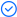WPS Office

Free All-in-One Office Suite with PDF EditorRead, edit, and convert PDFs with the powerful PDF toolkit.Microsoft-like interface, easy to use.

Windows • MacOS • Linux • iOS • Android# How to check if a cell contains text in Excel

August 1, 2022
4.2K Views

You may use your data in helpful ways with the help of a number of Excel formulae. You could, for instance, receive a result based on whether or not a cell satisfies a set of requirements. We'll concentrate on functions that indicate if a cell has text for the time being.

Containing Specific Text

Use Excel's ISNUMBER and SEARCH functions to see if a cell contains a specific piece of text. Excel lacks a CONTAINS function.

1.  Use the SEARCH function to locate a substring's location inside a text string.

Explanation: Excel appears at position 17, text appears at position 17, and cell A2 does not contain the words store, formula, or combine, which are found at positions 12 and 6, respectively.

2.   ISNUMBER function should be added. If a cell contains a number, the ISNUMBER function returns TRUE; otherwise, it returns FALSE.

The term excel is present in cell A1, store is absent from cell A2, text is present in cell A3, and combine is present in cell A4.

3.   Without displaying the substring, you can also see if a cell includes a particular piece of text. The substring must be enclosed in double quotation marks.

4.   Use the FIND function instead of the SEARCH function to conduct a case-sensitive search instead.

The term excel is present in cell A1, store is absent from cell A2, text is present in cell A3, and combine is present in cell A4.

5.   Introduce the IF function. If a cell contains particular text, the following formula (which is case-insensitive) yields Found, else it returns Not Found.

Why cell C2's formula becomes =IF(COUNTIF(A2,*store*),Found”, “Not Found). A series of zero or more characters is identified by an asterisk (*).

# If Cell Contains Text Then SUM

The Excel formula to sum whether a cell has text is shown below. If a particular string is present in the cell, you can sum its values. Here is an illustration of how to add the values in column B based on the values in another column.

If the specified text appears in any of the cells in Column A, the formula will sum the values in Column B.

# If Cell contains text from the list then return value

This Excel formula determines whether a cell has text from a list before returning the value. To check the array of values in a Cell and return the specified value, we can use the COUNTIF and OR functions. The following formula checks the list in cells D2 to D5, checks Cell A2, and returns the value to Cell B2.

= If (Return Value, ), OR (COUNTIF(A2,*&\$D\$2: \$D\$5&*)), )

Excel will assign a value if a cell includes a word.

The formula below can be changed to verify if a word is present in the cell before assigning a value.

=IFERROR(IF(SEARCH(“Word”,A2,1)>0,1,0),””)

A specified word will be checked for in the needed cell using the search function, and its location will be returned. If the value is larger than 0, we can use the If function to assign a specific value (for instance, 1) to the cell. The search feature yields #Value the IFERROR function can be used to handle the situation if no match was found in the cell.

Did you learn how to determine whether a cell in Excel includes text? To discover more about the functionality of Word documents, Excel spreadsheets, and PowerPoint slides, follow WPS Academy. To edit word, excel, and PowerPoint documents for free, you may also download WPS Office. Get it now! and have a pleasant and straightforward working experience.